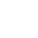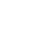LV. 50
GP 8k^^^^^^^^^^^^^^^^^^^^^^

(諸神進入英靈殿)

^^^^^^^^^^^^^^^^^^^^^^^^^

(作者華格納晚年的側像)

B0%BC%E5%B8%83%E9%BE%8D%E6%A0%B9%E7%9A%84%E6%8C%87%E7%92%B0%E3%80%8B

Festspielhaus，這所劇場也成為專演華格納作品的場地，至今還每年舉辦Bayreuther
Festspiele音樂節。上文說看過的舊DVD便是1988年Bayreuther Festspiele的演出了

^^^^^^^^^^^^^^^^^^^^^^^^^^

(套裝包裝)

Salminen)建造新的城堡後，巨人要情愛女神芙蕾婭(Sabina von Walther)獻身給他們，事後女神拒絕，而其他女神Fricka(Anna Larsson)和Erda(Christa Mayer)反口，巨人便要挑戰雷神索爾(Ilya Bannik)與和平神弗雷(German Villar)。火神洛吉(John Daszak)提議用同等價值的，萊茵的黃金代替女神的愛，便去搶尼布龍的指環。

ALBERICH的弟弟MIME(Gerhard Siegel)被兄長控制著，但仍然有意志的，而神明到尼布龍根深淵，說要解放他當然要把指環弄出來。

^^^^^^^^^^^^^^^^^^^

^^^^^^^^^^^^^^^^^^^^

(女神身後的穿上金黃衣服的La Fura Dels Baus舞蹈員們)

^^^^^^^^^^^^^^^^^^^^^^

LV. 50
GP 8k^^^^^^^^^^^^^^^^^^^^^^^^^^^^^^^^^^^^^^^^^^

^^^^^^^^^^^^^^^^^^

La Fura Dels Baus做出人肉火圈

^^^^^^^^^^^^^^^^^^^^^^^^^^^^^^

The Elder Edda神話原著指環本身沒有力量，只是被搶的人要詛咒邪神洛奇要接受報應，後來洛奇被奧丁徵罰到世界末日為止，但那是洛奇而不是本編的火神洛吉。

^^^^^^^^^^^^^^^^^^^

^^^^^^^^^^^^^^^^^^^^^^^^^^^^^^^^^^^^^^^^^^^

Festspiele音樂節1988時換裝，但DVD是在看圖書館看的無法截圖，網絡只找到這幅的圖。

^^^^^^^^^^^^^^^^

LV. 50
GP 8k3 樓 yau gx9900gundam
GP0 BP-

^^^^^^^^^^^^^^^^^^^^^^^^^^^^^^^^^^^^^

https://home.gamer.com.tw/creationDetail.php?sn=4562118

https://forum.gamer.com.tw/C.php?bsn=60229&snA=10608&tnum=1

^^^^^^^^^^^^^^^^^^^^

^^^^^^^^^^^^^^^^^^^^^^^^^^^^^^

(本集碟版的封面)

^^^^^^^^^^^^^^^^^^^^^^^^^^^^^^^

Marina Zyatkov扮演的「鳥人」，卻在本編的服裝中較特別搶眼的。

LV. 50
GP 8k## 梅塔(Z.MEHTA)版華格納的尼布龍根的指環 四 諸神的黃昏(大結局)

^^^^^^^^^^^^^^^^^

^^^^^^^^^^^^^^^^^^^^^^^^^^^^^^^^^^^^^^

()號內是名伶的名字。

bd封面

^^^^^^^^^^^^^

(顧德爾的造型)

^^^^^^^^^^^^^^^^^^^

*不論是費爾森家的薩加或尼布龍根之歌，哈根都只是勃艮第的將軍而不是王子。

^^^^^^^^^^^^^^^^^^^^^^^^^^^^^^

^^^^^^^^^^^^^^^^^^^^^^^^^^^^^^^^^^^

^^^^^^^^^^^^

face基於日前微軟官方表示 Internet Explorer 不再支援新的網路標準，可能無法使用新的應用程式來呈現網站內容，在瀏覽器支援度及網站安全性的雙重考量下，為了讓巴友們有更好的使用體驗，巴哈姆特即將於 2019年9月2日 停止支援 Internet Explorer 瀏覽器的頁面呈現和功能。